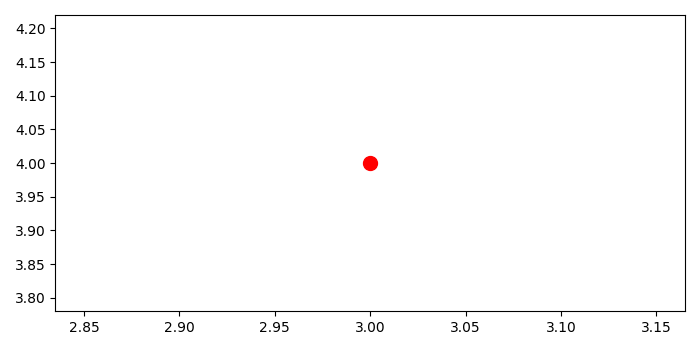# Set variable point size in Matplotlib

MatplotlibServer Side ProgrammingProgramming

#### Python Data Science basics with Numpy, Pandas and Matplotlib

Most Popular

63 Lectures 6 hours

#### Data Visualization using MatPlotLib & Seaborn

11 Lectures 4 hours

#### MatPlotLib with Python

9 Lectures 2.5 hours

To set the variable point size in matplotlib, we can take the following steps−

• Initialize the coordinates of the point.
• Make a variable to store the point size.
• Plot the point using scatter method, with marker=o, color=red, s=point_size.To display the figure, use show() method.

## Example

from matplotlib import pyplot as plt
plt.rcParams["figure.figsize"] = [7.00, 3.50]
plt.rcParams["figure.autolayout"] = True
xy = (3, 4)
point_size = 100
plt.scatter(x=xy, y=xy, marker='o', c='red', s=point_size)
plt.show()

## Output